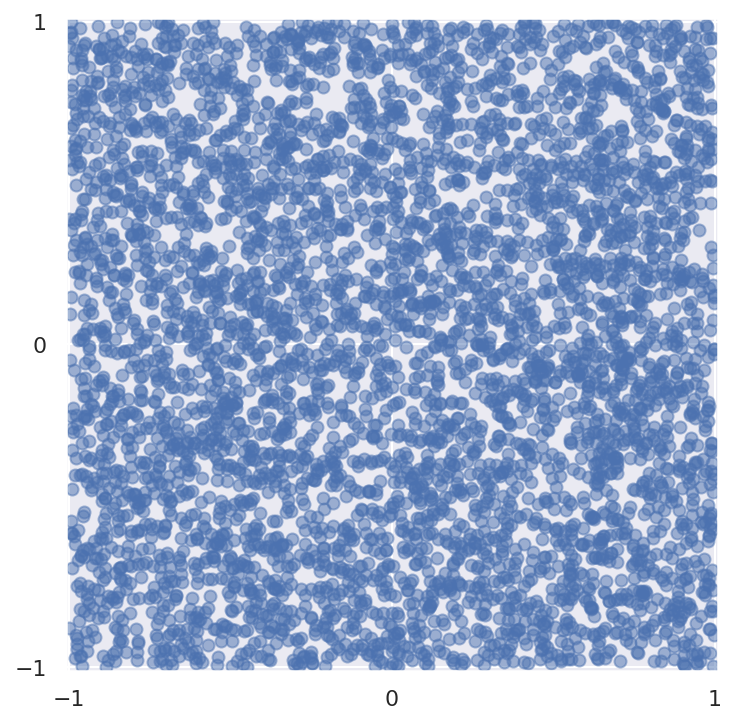# A Modern Introduction to Probabilistic Programming with PyMCI first started working with probabilistic programming about ten years ago (in late 2012 or early 2013) using PyMC2. At the time I was preparing to leave a PhD program in pure math for a data science career in industry. I found that the Bayesian approach to statistics and machine learning appealed to my mathematical sensibilities. I soon found PyMC3 and loved how it provided a fast path to translate the mathematical models I had in my head into executable code. A lot has changed in the past ten years; I have grown professionally and technically, the theory behind applied Bayesian inference (in particular adaptive Hamiltonian Monte Carlo algorithms) has blossomed, and PyMC and its related libraries have matured. This post presents an introduction to what I think of as a modern probabilistic programming with PyMC from a perspective that my experience over the last decade has shaped. It is modern in two respects:

1. It features modern Bayesian inference algorithms and best practices, preferring adaptive Hamiltonian Monte Carlo samplers over Metropolis-Hastings-style ones. It introduces recently developed/enhanced diagnostic tools to diagnose the convergence (or not) of these agorithms.
2. It features the cutting-edge beta version of PyMC v4 and relies heavily on Aesara and ArviZ, both of which did not exist ten years ago.

This post is an extended version of an introductory talk I gave in January 2022 for the Data Umbrella and PyMC sprint meant to introduce potential new contributors to PyMC. You can find a video of this talk on YouTube.

First we make the necessary Python imports and do some light housekeeping.

In :
%matplotlib inline
%config InlineBackend.figure_format = 'retina'

In :
from warnings import filterwarnings

In :
from aesara import pprint
from matplotlib import pyplot as plt, ticker
import numpy as np
import pandas as pd
import scipy as sp
import seaborn as sns
from sklearn.preprocessing import StandardScaler

In :
filterwarnings('ignore', category=RuntimeWarning, message="overflow encountered in exp")
filterwarnings('ignore', category=UserWarning, module='pymc',
message="Unable to validate shapes: Cannot sample from flat variable")

In :
FIG_SIZE = np.array([8, 6])
plt.rc('figure', figsize=FIG_SIZE)

dollar_formatter = ticker.StrMethodFormatter("${x:,.2f}") pct_formatter = ticker.StrMethodFormatter("{x:.1%}") sns.set(color_codes=True)  ### Probabilistic programming from three perspectives¶ In this section we motivate probabilistic programming from three perspectives: theoretical, statistical, and computational. #### Theoretical¶ There is a pervasive perspective (MIT Sloan, Forbes, storytellingwithdata.com provide just a few examples) that data science and analytics involves collecting, storing, and analyzing data to produce a story about the world that is compelling enough to change the behavior of individuals, businesses, or systems. As with all sufficiently popular perspectives this is not incorrect, but the relationship between the data and storytelling warrants closer examiniation. Consider the following diagram, Charles Joseph Minard's famous map of Napoleon's Russian campaign.Edward Tufte, one of the foremost authorities on the subject of data visualization, considers that "[this] may well be the best statistical graphic ever drawn" in his classic book The Visual Display of Quantitative Information (p 40). This figure certainly uses data (the size of Napoleon's army at various points in time, distance covered, temperature, and more) to tell a compelling story about the perils of invading Russia. In this case, the data leave very little room for differences of interpretation (although it leaves plenty of opportunity for beautiful design). Almost no data set we encounter in our daily work will ever tell a story as obviously as this one does. For me, the central theoretical insight of probabilistic programming is that, given the uncertainty and ambiguity inherent in most data sets, it is more productive to start with stories of how the data might have been generated, then use the observed data to reason about those stories. #### Statistical¶ This discussion is quite abstract; we now use the language of statistics to make it more concrete. Rephrased in terms of conditional probability, the popular perspective that a story flows naturally from the data is analagous to searching for a story such that conditional probability$P(\text{Story}\ |\ \text{Data})$(the probability of the story given the data) is quite high. The central insight of probabilistic programming above says that this search is much easier if instead we begin by telling stories of how the data may have been generated, which is analagous to specifying$P(\text{Data}\ |\ \text{Story})$. Having specified$P(\text{Data}\ |\ \text{Story})$, how can we then reverse this conditional probabiltiy to arrive at$P(\text{Story}\ |\ \text{Data})$? The most straightforward answer is to use Bayes' theorem.Recast in our notation, Bayes' theorem becomes $$P(\text{Story}\ |\ \text{Data}) = \frac{P(\text{Data}\ |\ \text{Story}) \cdot P(\text{Story})}{P(\text{Data})}.$$ Allowing$\mathcal{D}$to denote our data and$\theta$to denote the unknown parameters of our data generating process we get a form of Bayes' theorem that is familiar to statisticians: $$P(\theta\ |\ \mathcal{D}) = \frac{P(\mathcal{D}\ |\ \theta) \cdot P(\theta)}{P(\mathcal{D})}.$$ The denominator of this expression, the marginal probability of the data, is calculated as $$P(\mathcal{D}) = \int P(\mathcal{D}\ |\ \theta) \cdot P(\theta)\ d\theta,$$ which leads to our third perspective on probabilistic programming. #### Computational¶ For many realistic models (as we will call stories about how our data was generated from now on), this integral for$P(\mathcal{D})$is analytically intractible and must be approximated. The most common approach taken to approximate this integral in probabilistic programming is through Monte Carlo methods. Monte Carlo methods use well-crafted sequences of random numbers to approximate integrals. The following basic example illustrates the basic idea behind Monte Carlo methods. ##### A Monte Carlo approximation of$\pi$¶ Generate 5,000 random points uniformly distributed in the square$-1, \leq x, y \leq 1$. In : SEED = 123456789 # for reproducibility rng = np.random.default_rng(SEED)  In : N = 5_000 x, y = rng.uniform(-1, 1, size=(2, N))  In : fig, ax = plt.subplots(subplot_kw={'aspect': 'equal'}) ax.scatter(x, y, alpha=0.5); ax.set_xticks([-1, 0, 1]); ax.set_xlim(-1.01, 1.01); ax.set_yticks([-1, 0, 1]); ax.set_ylim(-1.01, 1.01);Consider the points that fall inside the unit circle centered at the origin,$x^2 + y^2 < 1\$.

In :
in_circle = x**2 + y**2 < 1

In :
fig, ax = plt.subplots(subplot_kw={'aspect': 'equal'})

ax.scatter(x[~in_circle], y[~in_circle], c='C1', alpha=0.5);
ax.scatter(x[in_circle], y[in_circle], c='C2', alpha=0.5);